## Questions1. A man bakes two types of cakes, queen cakes and marble cakes. Each week he bakes queen cakes and y marble cakes. The number of cakes baked are subject to the following
conditions; 30
x + 20y 4800, 30x + 40y 3600 and 10x >30y. He makes a profit of shs.10 on each queen cake and shs.12 on each marble cake.
1. Draw a graph to represent the above information on the grid provided
2. From the graph, determine how many cakes of each type he should make to maximize his weekly profit
3. Calculate the maximum profit
4. If he is to make a weekly profit of at least shs.600, find the least number of marble cakes he should bake
2.  A company produces shirts and jerseys using two types of machines. Every shirt made requires 2 hours on machine A and 2 hours on machine B. Every Jersey made requires 3hours on machine A and I hour on machine B. In one day the time limit on machine is 24hours but that on machine B is 12hrs. The number of Jerseys produced must not be more than the shirts produced in one day. The company makes a profit of shs.200 on each shirt and shs.200 on each Jersey. The company produces x shirts and y jerseys per day
1. Write down four inequalities which must be satisfied by x and y and represent these inequalities on a grid
2. Find the values of x and y which will give the company maximum daily profit and also state the maximum profit
3.  A trader makes two types of chair, ordinary and special chairs. The cost of each ordinary chair is shs.300 while each special chair costs shs.700. He is prepared to spend not more than shs.21,000. It is not viable for hi m to make less than 20 chairs. Ordinary chairs must be less than twice the special chairs but more than 15. By taking the number of ordinary chairs as and special chairs as y:
1. Write down all the inequalities in x and y
2. Draw the inequalities on the grid provided
3. He sells a special chair at a profit of shs.140 while ordinary chairs at a profit of shs.120; Determine the maximum possible profit
4. A school has to take 384 people for a tour. There are two types of buses available.
Type X and type Y. Type X can carry 64 passengers and type Y can carry 48 passengers. They have to use at least 7 buses.
1. Form all linear inequalities which will represent the above information .
2. On the grid provided, draw the inequalities and shade the un-wanted region.
3. The charges for hiring the buses are ;
Type X: shs.25,000
Type Y: shs.20,000
Use your graph to determine the number of buses of each type that should be hired to minimize the cost.
5. A shoe maker makes two types of shoes A and B. He takes 3 hours to make one pair of type A and 4 hours to make one pair of type B. He works for a maximum of 120 hours to make pairs of type A and y pairs of type B. It costs him Kshs. 400 to make a pair of type A and Kshs.150 to make a pair of type B. His total cost does not exceed kshs.9000. He must make at least 8 pairs of type A and 12 pairs of type B.
1. Write down four inequalities representing the information above
2. On the grid provided represent the inequalities and shade the unwanted regions
3. The shoe maker makes a profit of kshs.40 on each pair of type A and kshs.70 on each pair
6. A theatre has a seating capacity of 250 people. The charges are shs.100 for an ordinary seat and shs.160 for a special seat. It costs shs.16,000 to stage a show and the threatre must make a profit. There is never more than 200 ordinary seats and for a show to take place at least 50 ordinary chairs must be occupied. The number of special seats is always less than twice the number of ordinary seats.
1. taking x to be the number of ordinary seats and y the number of special seats, write down all the inequalities representing the information above.
2. On the grid provided, draw the graph to show the inequalities in (a) above
3. Determine the number of seats of each type that should be booked in order to maximize the profit.
7. A man sells two types of ice creams in cups and sticks. He can store less than ten packets in his cooling box. He sells more cups than sticks but less than 3 items as many cups as sticks. He also knows that he will sell more than 3 packets of sticks. His profit is shs.3.00 on a packet of cups and shs.2.00 on a packet of sticks.
1. Form inequalities to represent the above information:
(Let x – packets of cups and
y – packets of sticks)
2. On the grid provided graph the inequalities to satisfy the required condition
3. How many packets of cups and sticks should the man put in his box to give him the highest profit?
8. A shopkeeper bought 50 pangas and 30 jembes :-
1. From a wholesalers for shs.4,260. He had bought half as many jembes and 5 pangas less, he would have paid shs.1290 less. Had the shopkeeper bought form wholesaler B, he would have paid 10% more a panga and 15% less for a jembe. How much would he have saved if he had bought the 50pangas and 30 jembes from wholesalers B
2. The price of a suit if marked at shs.5000. A discount
9. The games master whishes to hire two matatus for a trip. The operators have a Toyota which carries 10passengers and a Kombi which carries 20 passengers. Altogether 120 people have to travel. The operators have only 20litres of fuel and the Toyota consumes 4 litres on each round trip and the Kombi 1 litre on each round trip. If the Toyota makes round trips and the kombi y round trips;
1. write down four inequalities in x and y which must be satisfied
2. represent the inequalities graphically on the grid provided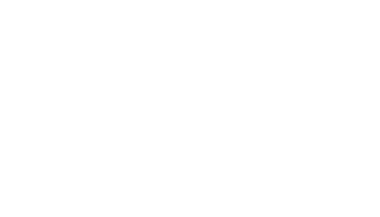3. The operators charge shs.100 for each round trip in the Toyota and shs.300 for each round trip in the kombi;
1. determine the number of trips made by each vehicle so as to make the total cost a Minimum
2. find the minimum cost
10. The velocity of a particle Vm/s moving in a straight line after t seconds is given by V = 3t2 – 3t – 6. Find the distance covered by the p1.
1. 30x + 20y ≤4800……..(i)
30x + 40y ≥
3600……..(ii)
10x <
30 y…………(iii)
x>
0 y >0
objective function 10 x+ 12 y = K
3x + 2y = 480          3x + 4y = 360           x = 3y
 X 40 60 80 X 20 40 60 X 30 45 60 y 180 150 120 Y 75 60 45 Y 10 15 20
2. consider (60,40)
10 (60) + 12(40) = 600 +480
=1080
10x + 12y = 108 0
5x + 6y = 540 – search line
 X 20 40 60 y 73 57 40
Maximum profit at ( α, 240)
No queen cake , 240 marble cakes
3. 240 X 12 = sh. 2880
4. 10x + 12y ≥600 ⇒10x + 12y = 600
5x + 6y = 300
 X α 12 60 y 50 40 0
2. Machine A                                                      Machine B
Shirts               Jerseys                              Shirts               Jerseys
No.  x                    y                                           x                     y
Hrs. @2hrs            @3hrs                                   @2hrs              @1hr
1. 2x + 3y ≤24
2. 2x + y ≤12
3.  y > x
4. x > 0
y > 0
Max pt(3,6)
Max profit = 22 x 3 + 200 x 6
= 600 + 1200
= Shs. 1800
(i) 2x + 3y =24
 X 0 12 y 8 0
(ii)2x+y =12
 X 0 6 y 12 0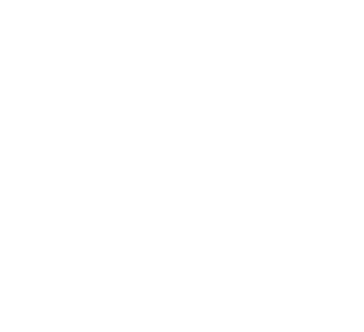3.
1. 3x + 7y ≤210
x + y 20
x < 2y
x > 15
2. refer
3. 120x + 140y = 120 x 130 + 140 x 10
Profit = shs.5960
x = 31
y = 16
4. Passengers
64x + 48y ≥ 384 i.e. 8x + 6y ≥ 48
x > 0
y > 0
x + y ≥ 7
Cost equation
Total cost = 2500x + 20000y
(3,4)
3 type x
4 type y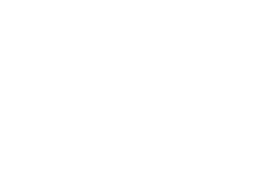5.
1. 3x + 4y 120
400x + 150y 9000
x 8       y >12
2. i) 3x + 4y ≤ 20
(ii) 40x + 15y ≥ 900
(iii) x ≥ 8
(iv) y > 12
(table showing calculation of profit)
 Points Objective function 40x + 70y Profit (i) (8,24) 320 + 1680 2000 (ii) (24, 12) 960 + 840 1800 (iii) (8, 12) 320 + 840 1160
3. 12 type x
12 type y
Profit = 40(24) + 70(12)
= 1800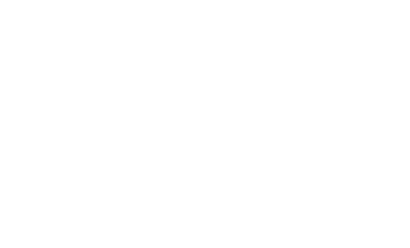6. 100 x = 160y = 16000                        5 x 200 + 8 x 50
=100 x 200 + 160 x 50                       1000 + 4000
20000 + 8000                                    10 x 200 + 16 x 50=
28000/=                                            10x + 16y = 1600
5x + 8y = 800
5 x 20 + 100
8y = 800 – 100
y = 700
8
800/5 = 160
1. y<2x, 50≤x≤200 x >100
y >0, x+y ≤250, 100x + 160y ≥ 16000
2. See graph
3. see graph
profit?
7.
1. x + y <10
y <3x
y > 3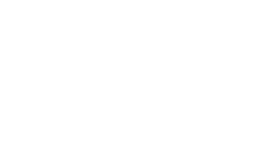2.
3. Obejctive function 3x = 2y = I or use of serach line 5 packets of cups and 4packets of stucks
 x y Profit 2 2 3 3 3 4 4 5 4 5 4 5 6 45 4 1416171921202223
8. Panga – P, Jembe J
1. 50P + 30J = 4260
50P + 15J = 1290
50P + 30J = 4260
10P + 30J = 1290
40P = 1680
P = 168 = 42
4
50(42) + 30J = 4260
2100 + 30J = 4260
30J = 2160
J = (2160)
30
J = 72
Wholesaler
110 x 42 = shs.46.50 = pangas
100
85  x 72 = shs 60 = jembes
100
For B
50 x 46.50 + 30 x 61.2
2310 + 1836 = 4146
Saving = 4260 – 4116 = 144
2. Discount 5000 – 3500 = 1500
% discount = 1500 x 100
5000
= 30%
9.
1. X 0, y = ≥ 0
10x + 20y ≥ 120
4x + y ≥ 20
2. On the graph.
1. (4,4)
4 x 100 + 4 x 300
400 + 1200 = 1600
10.  Distance Covered = (3t2 – 3t -6)dt
= t3 3/2 t2 – 6t44
{43 3/2(4)2– 6(4)} - {13 – 3(1)2 – 6(1)}
16 - -13/2= 22.5